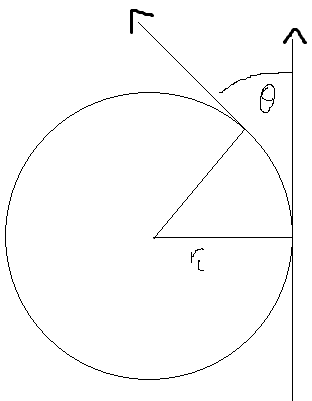# Cosmic Rays and Larmor Radius

SHISHKABOB

## Homework Statement

Calculate the minimum energy of a cosmic ray that it needs to have in order to "point" back to its origin. Let's define this as being deflected by the Galactic magnetic field of B = 1μG by less than 1°, as it traverses a distance of d = 1 kpc within the Galaxy. Calculate this for a proton and an iron nucleus.

rL = E/qB

## The Attempt at a Solution

so it's a pretty straightforward question, but where I've hit trouble is trying to figure out the larmor radius.

The original vector of the particle is a line tangent to a circle with radius rl. Would the 1 kpc be the arc length along that circle that the particle travels along? If so, then I should be able to use some geometry to relate the angle that the particle is deflected to the larmor radius, right?

voko
Calculate the minimum energy of a cosmic ray that it needs to have in order to "point" back to its origin. Let's define this as being deflected by the Galactic magnetic field of B = 1μG by less than 1°, as it traverses a distance of d = 1 kpc within the Galaxy.

The statement is confusing. On the one hand, it talks about "pointing back". On the other hand, "being deflected less than 1 degree". What does that really mean?

SHISHKABOB
the cosmic ray particle is deflected from its original path by some magnetic field so that it forms part of a circle with radius rL, the larmor radius

so by comparing the original path with the deflected path, you can find the angle between them. The question is asking for some minimum energy to cause this larmor radius. By looking at the cosmic ray, we see it coming from its deflected path, but really it came from some other direction and was deflected by a magnetic field. If the energy is high enough, then the deflection will be minor and so the direction that it appears to come from will be similar to the direction that it really came from.

Basically, I just need to figure out the relationship between rL and the angle theta in this drawing:but I'm stumped by the geometry :(

voko
So I guess we can ignore the part on "pointing back". I interpret this thusly: the particle goes through an arc of a certain circle, the length of the arc given, and the vectors of the initial and final velocity are within one degree. Does this help in any way?

SHISHKABOB
yes, I think I've figured it out

and yeah, the "pointing back" part is just there to tie the problem back to observational astrophysics, because the class is an astronomy class